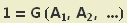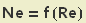## Monday, 4 June 2007

### Dimensionless

In my life there are no pretty pictures or pretty colors but how about some pretty maths?

Chemical engineering, which is my current lab, is much too applied for me but I do like the maths behind it. This is an example of why it is useful to use dimensionless quantities. The prove comes from myself but I don't think it's wrong. For more information you can check out information on the Buckingham π theorem.

We assume that for any stirrer type the power (P) needed to run a stirrer depends only on the diameter (d), the rotational frequency (n), the density (ρ) and the dynamic viscosity (η).We can divide by P and get rid of the dimensions on both sides.Since the right side has no dimension one has to be able to rewrite it using dimensionless quantities Ai (or the dimensions wouldn't cancel out).Because only multiplication and exponentiation changes the unit, we can consider only Ai of the following form without loss of generality  (the cA are rational numbers).The quantities are made up of three units: kg, m, s. If we want Ai to be without dimension, all three units have to cancel out. This comes down to a homogenous linear equation system.The solution is a 2-dimensional space.We just have to pick 2 dimensionless quantities (let's call them Ne and Re) and all the others can be made up as linear combinations (in our case that means multiplication and exponentiation). The equation can be rewritten as (with a 2 variable function H)We were interested in the power. That means one quantity shouldn't include P. Then only one solution (a 1-dimensional subspace) remains. We choose the smallest whole coefficients and get the Reynolds number.The other number we choose is the Newton number (there is no strict reason why we took it).Since we were able to explicitely express P we can also express Ne and we get a function f of one variable.Now we can start experimenting. We only have to determine the function f for one stirrer size, frequency, medium density and viscosity. Then we can change any of the parameters and know what happens. The problem is that the initial assumption was only a simplification and we have more parameters. That's why it's not quite as nice.

Anyway this is a pretty cool example of how a little bit of abstract basic mathematics can save a lot of work. 1 hour at your desk doing maths can save you 2 weeks experimenting. Or how does that saying go?

 If you want mathematicians to like you, you have to say "without loss of generality" as much as possible.